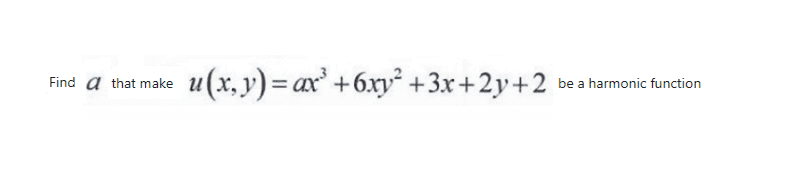# Harmonic function problem

• MHB
• jaychay

#### jaychayIn this case, to be Harmonic, you would need \displaystyle \begin{align*} \frac{\partial ^2 u}{\partial x^2} + \frac{\partial ^2 u}{\partial y^2} = 0 \end{align*}...
In this case, to be Harmonic, you would need \displaystyle \begin{align*} \frac{\partial ^2 u}{\partial x^2} + \frac{\partial ^2 u}{\partial y^2} = 0 \end{align*}...